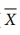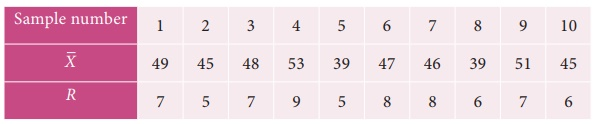Home | | Business Maths 12th Std | Exercise 9.3: Statistical Quality Control (SQC)

# Exercise 9.3: Statistical Quality Control (SQC)

Book back answers and solution for Exercise questions - Maths: Applied Statistics: Statistical Quality Control (SQC): Problem Questions with Answer, Solution

Exercise 9.3

1. Define Statistical Quality Control.

2. Mention the types of causes for variation in a production process.

3. Define Chance Cause.

4. Define Assignable Cause.

5. What do you mean by product control?

6. What do you mean by process control?

7. Define a control chart.

8. Name the control charts for variables.

9. Define mean chart.

10. Define R Chart.

11. What are the uses of statistical quality control?

12. Write the control limits for the mean chart.

13. Write the control limits for the R chart.

14. A machine is set to deliver packets of a given weight. Ten samples of size five each were recorded. Below are given relevant data:Calculate the control limits for mean chart and the range chart and then comment on the state of control. (conversion factors for n = 5, A2 = 0.58 , D3 = 0 and D4 = 2.115 )

15. Ten samples each of size five are drawn at regular intervals from a manufacturing process. The sample means () and their ranges ( R ) are given below:Calculate the control limits in respect ofchart. ( Given A2  = 0.58 , D3  = 0 and D4  = 2.115 )

Comment on the state of control.

16. Constructand R charts for the following data:(Given for n = 3, A2 = 0.58 , D3 = 0 and D4 = 2.115 )

17. The following data show the values of sample mean () and its range ( R ) for the samples of size five each. Calculate the values for control limits for mean , range chart and determine whether the process is in control.( conversion factors for n = 5, A2 = 0.58 , D3 = 0 and D4 = 2.115 )

18. A quality control inspector has taken ten samples of size four packets each from a potato chips company.The contents of the sample are given below, Calculate the control limits for mean and range chart.(Given for n = 5, A2 = 0.58 , D3 = 0 and D4 = 2.115 )

19. The following data show the values of sample means and the ranges for ten samples of size 4 each. Construct the control chart for mean and range chart and determine whether the process is in control.20. In a production process, eight samples of size 4 are collected and their means and ranges are given below. Construct mean chart and range chart with control limits.21. In a certain bottling industry the quality control inspector recorded the weight of each of the 5 bottles selected at random during each hour of four hours in the morning.